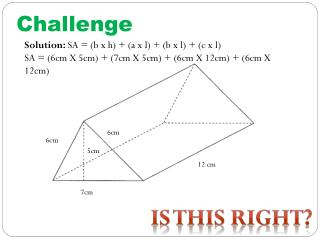DownloadDownload PresentationChallenge

# Challenge

Télécharger la présentation## Challenge

- - - - - - - - - - - - - - - - - - - - - - - - - - - E N D - - - - - - - - - - - - - - - - - - - - - - - - - - -
##### Presentation Transcript

1. Challenge Solution: SA = (b x h) + (a x l) + (b x l) + (c x l) SA = (6cm X 5cm) + (7cm X 5cm) + (6cm X 12cm) + (6cm X 12cm) 6cm 6cm 5cm 12 cm 7cm Is this right?

2. Given: a = 7cm b = 8cm c = 11cm height = 7.5cm length = 9cm Required: Surface Area of Triangular Prism Analysis: SA = (b x h) + (a x l) + (b x l) + (c x l) Solution: SA = (8cm x 7.5cm) + (7cm x 8cm) + (8cm x 9cm) + (11cm x 9cm) = Paraphrase: Therefore the Surface Area of this Triangular Prism is 277 cm³ Your Turn……… 9 cm 7 cm 7.5 cm 11 cm 8 cm

3. Homework Check

4. Introduction to 3.4: Volume of Triangular Prism Lesson 11 – Friday November 18 100mins

5. Recall • Area of a triangle is 1/2 (base X height) Which is one half the area of a rectangle • To calculate Volume of a Rectangular prism Volume = _________ http://www.youtube.com/watch?v=g2UnCcmoabY&feature=fvsr http://www.mathsnet.net/geometry/solid/houses1.html

6. Activity • Number everyone 1 to 4 • Give students a net for a triangular prism • Students are to create a rectangular prism that would fit the triangular prism. • Come up with a way to calculate the volume of the triangular prism based on what you know about the area of a triangle and rectangle and volume of rectangular prism • You are not allowed to use your textbook for aid. • Write down your thoughts of how you came up with the formulas.

7. Define Volume: __________________________________________________________________________ Formula to calculate VOLUME Of a Triangular Prism

8. Class Example: Area of Base = ____m² Volume = ____m³

9. Class Example #2:

10. Homework: Page 119 – 120 # 1 - 6

11. Reminder: TEST: Wednesday November 23, 2011